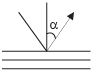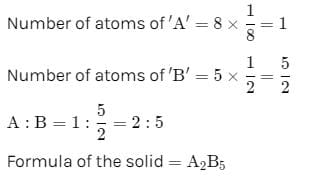# Test: Solid State - 2

## 30 Questions MCQ Test Physical Chemistry | Test: Solid State - 2

Description
Attempt Test: Solid State - 2 | 30 questions in 90 minutes | Mock test for Chemistry preparation | Free important questions MCQ to study Physical Chemistry for Chemistry Exam | Download free PDF with solutions
QUESTION: 1

Solution:
QUESTION: 2

### The density of CaF2 (fluorite structure) is 3.18 g/cm3. The length of the side of the unit cell is:

Solution:

d = zM/NA × V
For flourite structure, z = 4 and M = 78
3.18 = (4 × 78)/6.023 × 1023 × a3
a3 = 312/(6.023×1023 × 3.18)
a3 = 16.28 × 10-23
a = 546 pm

QUESTION: 3

### If the anions (A) form hexagonal closest packing and cations (C) occupy only 2/3 octahedral voids in it, then the general formula of the compound is

Solution:
QUESTION: 4

A solid is formed and it has three types of atoms X, Y, Z. X forms a FCC lattice with Y atoms occupying all the tetrahedral voids and Z atoms occupying half the octahedral voids. The formula of the solid is:

Solution:
QUESTION: 5

Which of the following will show Schottky defect?

Solution:

The Schottky defects are more common in ionic compounds with high coordination numbers and where the positive and negative ions are of similar size.

Examples include NaCl, CsCl, KCl, KBr and AgBr. Both Schottky and Frenkel defects are observed in AgBr.

Frenkel defects are observed in ZnS, AgCl, AgBr and AgI.

QUESTION: 6

In FCC unit cell, what fraction of edge is not covered by atoms:

Solution:
QUESTION: 7

If edge length of the unit cell is 356 pm, then radius of carbon atom in diamond:

Solution:
QUESTION: 8

In a cubic unit cell, the angle between normal to the planes (111) and (121) is:

Solution:
QUESTION: 9

How many unit cells are present in a cube-shaped ideal crystal of NaCl of mass 1.0 g?

Solution:
QUESTION: 10

Co-ordination no. of ‘c’ in Be2C3 whose structure is co-related with CaF2 is:

Solution:
QUESTION: 11

The value of d111 in a cubic crystal is 325.6 pm. The value of d333 is (d = interplaner spacing):

Solution:
QUESTION: 12

X-rays of wKa (wavelength 154 pm) are diffracted by a set of atomic planes in a crystals in the following manner. The separation of the la yers in the crystals in 404 Pm. Find the angle a along which the first order reflection will occur:Solution:
QUESTION: 13

In Rocksalt structure:

Solution:
QUESTION: 14

In a fcc lattice, atom A occupies the corner position and atom B occupies the face centre position. If one atom of B is missing from one of the face centred points, the formula of the compound is:

Solution:QUESTION: 15

The angle between the two planes represented by the Miller indices (110) and (111) in a simple cubic:

Solution:

cosθ = (h1h2 + k1k2 + l1l2)/(h12 + k12 + l12)1/2(h22+ k22 + l22)1/2

=> (1+1+0)/[(12 + 12 + 02)1/2(12 + 12 + 02)½]

=> 2/(6)1/2

=> cos-1 2/(6)1/2

=> 30o approx.

QUESTION: 16

The coordinate number for an atom in a primitive cubic unit cell is

Solution:

The coordination number for an atom in a primitive cubic unit cell is 6. Each sphere present at a corner of the unit cell is surrounded by 6 neighboring spheres present at 6 other corners.

QUESTION: 17

The three dimensional graph of lattice points which sets the pattern for the whole lattice is called

Solution:
QUESTION: 18

When Frenkel defects are created in an otherwise perfect ionic crystal, the density of the ionic crystal:

Solution:
QUESTION: 19

The compound formed by elements X and Y crystallizes in cubic structure in which X atoms are at the corners of the cube and Y atoms are at the face centre . The formula of the compound is

Solution:
QUESTION: 20

The angle at which the first order Bragg reflection is observed from (110) plane in a simple cubic unit cell of side 3.238 Å when chromium Ka radiation of wavelength 2.29 Å is used, is:

Solution:
QUESTION: 21

Number of rotational symmetry axes for triclinic crystal system is:

Solution:
QUESTION: 22

The lattice parameter of an element stabilized in a fcc structure is 4.04 Å. The atomic radius of the element is:

Solution:
QUESTION: 23

A metal crystallizes in fcc structure with a unit cell side of 500 pm. If the density of the crystal is 1.33 g/cc, the molar mass of the metal is closed to:

Solution:
QUESTION: 24

Percentage of free space in a body centred cubic unit cell is

Solution:
QUESTION: 25

An element exists in two crystallographic modifications with FCC and BCC structures. The ratio of the densities of the FCC and BCC modifications in terms of the volumes of their unit cells (VFCC and VBCC) is:

Solution:
QUESTION: 26

Metallic gold crystallizes in fcc structure with unit cell dimension of 4.00 Å The atomic radius.

Solution:
QUESTION: 27

Both NaCl and KCl crystallize with Fcc structure. However, the X-ray powder diffraction pattern corresponds to the fcc structure whereas, that, of KCl corresponds to sample cubic structure. This is because:

Solution:
QUESTION: 28

In a simple cubic, body-centred cubic and face-centred cubic structure, the ratio of the number of atoms present is respectively

Solution:

Face centered cube consists of eight atoms at the corners of the cube and six atoms at the center of each face. Hence, the ratio of number of atoms present in a simple cubic, body centered cubic and face centered cubic structures is 1 : 2 : 4.

QUESTION: 29

The interplanar spacing of (110) planes in a cubic unit cell with lattice parameter a = 4.242 Å is:

Solution:
QUESTION: 30

The edge length of a face centred cubic cell of an ionic substance is 508 pm. If the radius of the cation is 110 pm, the radius of the anion is

Solution:Use Code STAYHOME200 and get INR 200 additional OFF Use Coupon Code